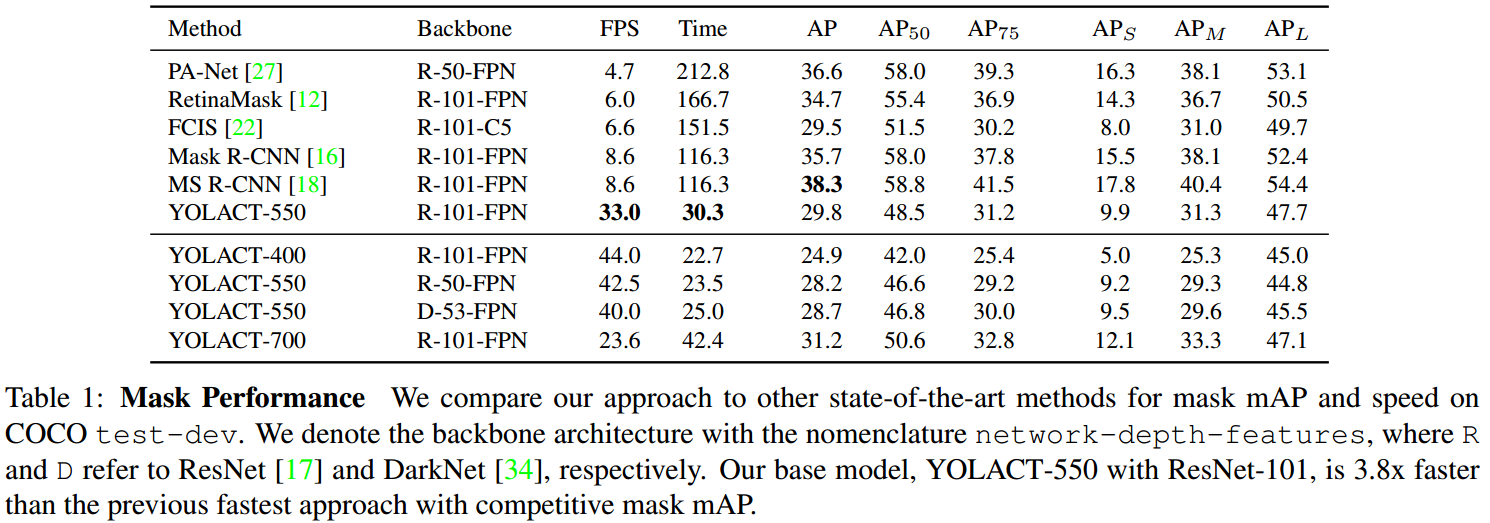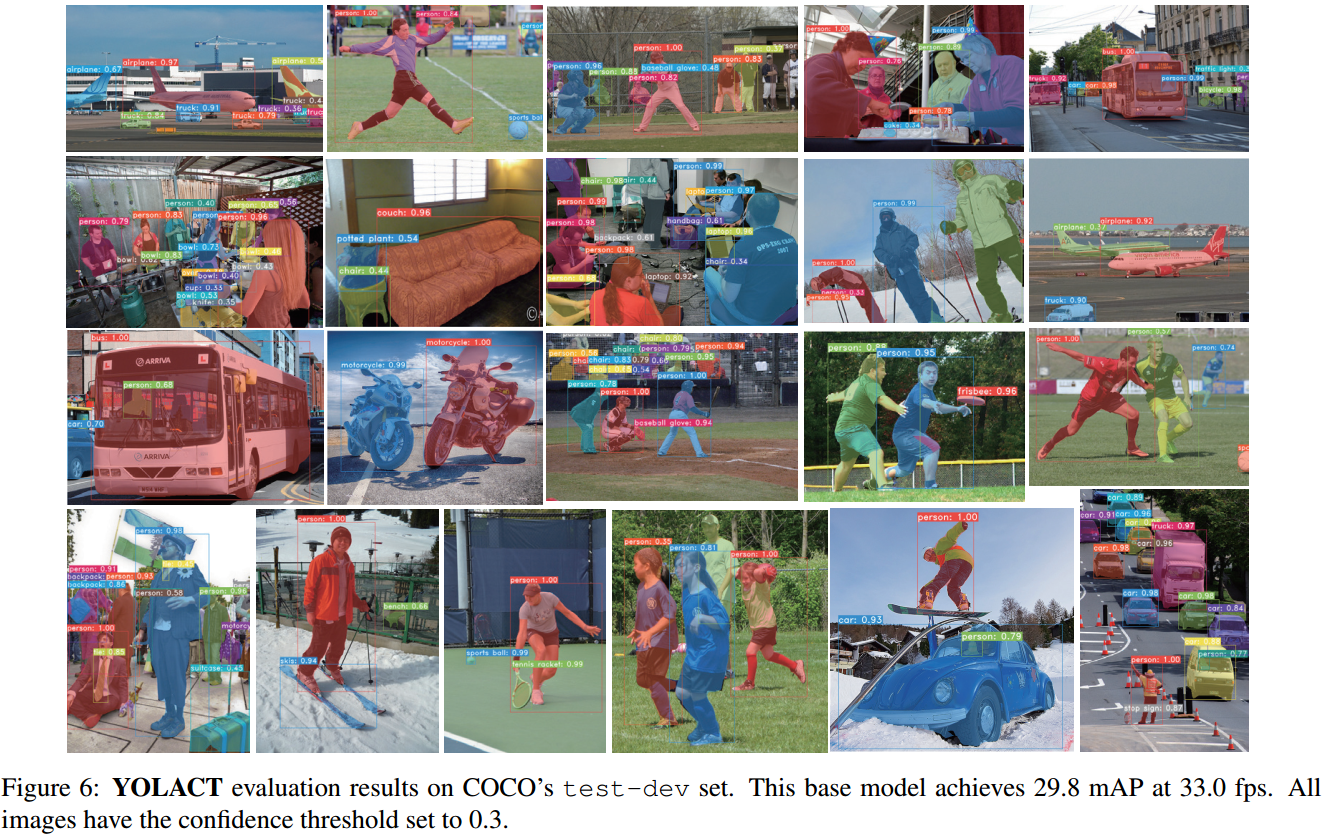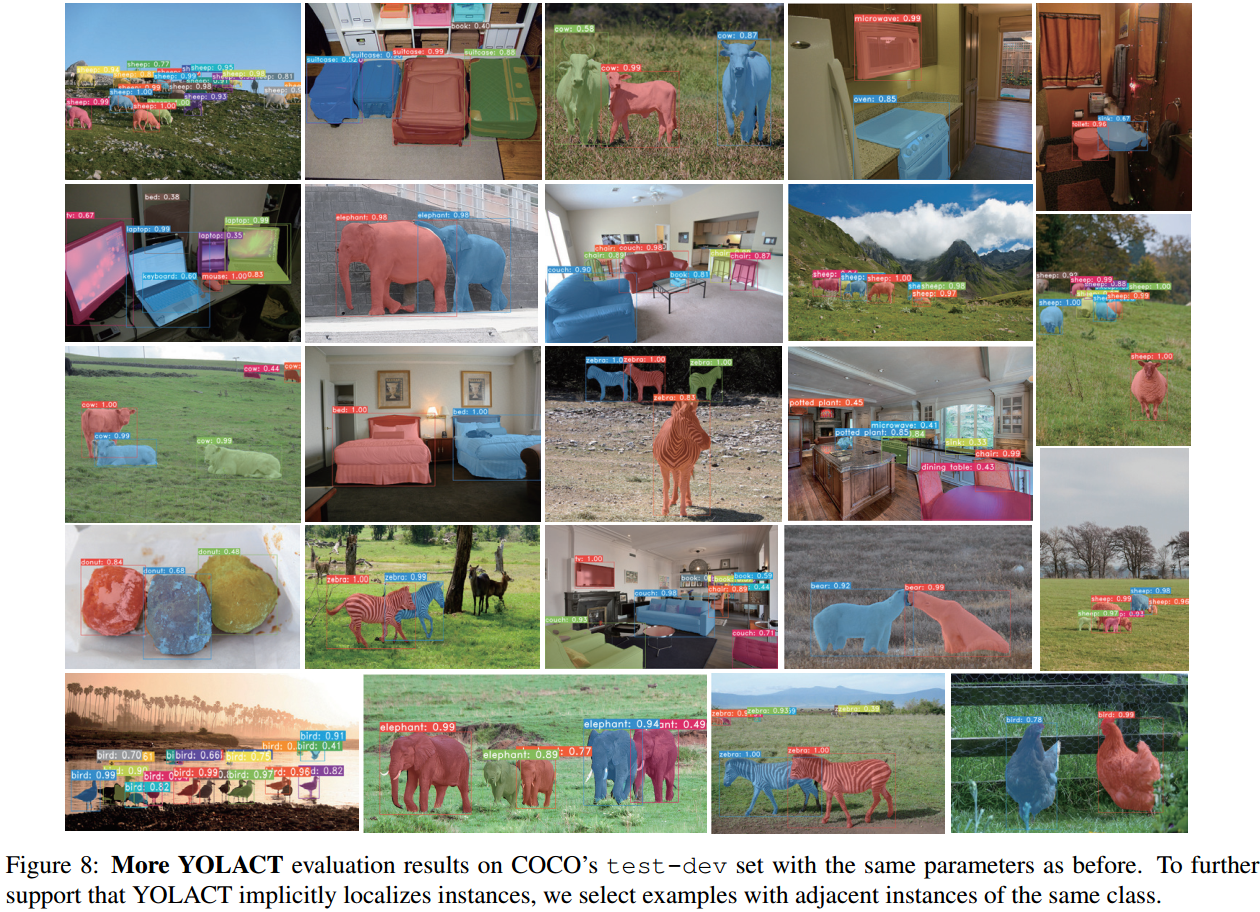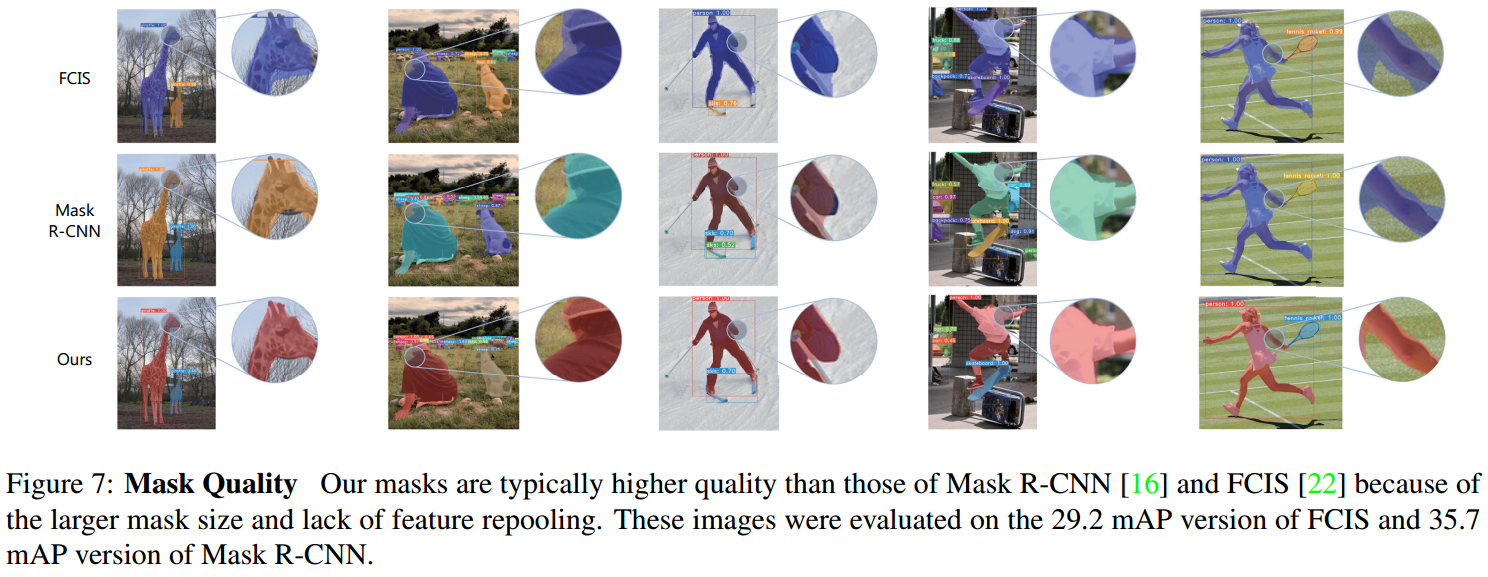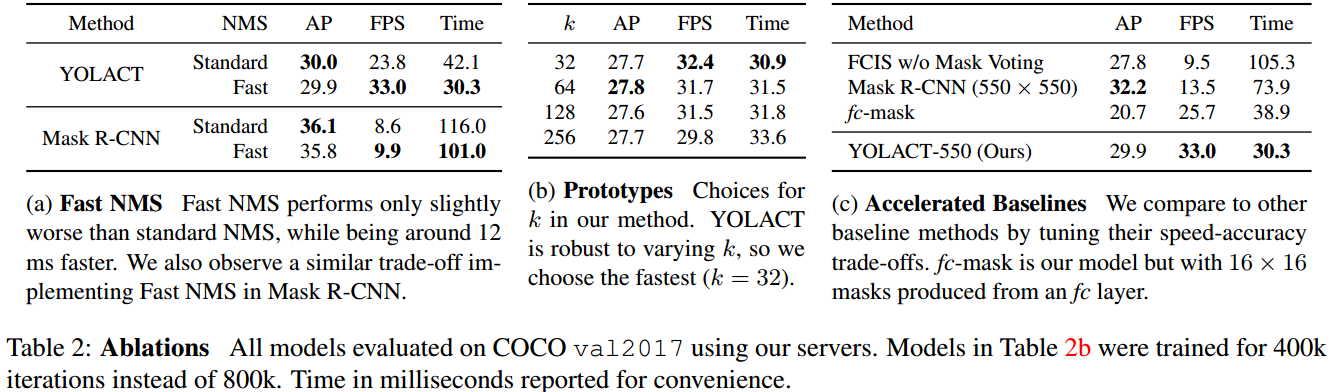YOLACT(You Only Look At CoefficienTs) 论文主要是提出了一种简单、快速的实时实例分割的全卷积模型，基于一块 Titan Xp 显卡，在 MS COCO 数据集上取得了 29.8 mAP 的精度，33fps 的速率. 此外，其仅是基于单张 GPU 训练得到的.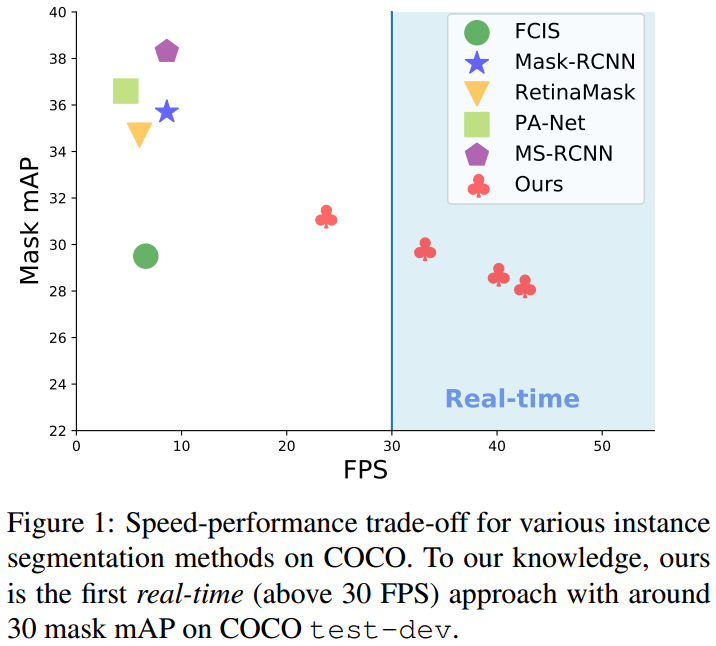Fig 1. 基于 COCO 数据集不同实例分割方法的速度与精度情况. YOLACT 第一次在 COCO test-dev 数据集取得了实时检测(大于 30 FPS)及约 30 的 mAP.

YOLACT 主要特点与贡献点：

 - 将实例分割划分为两个并行任务

• (1)生成整张图片的非局部非原型mask的字典 (generating a dictionary of non-local prototype masks over the entire image.)
• (2)预测每个实例的线性组合系数集合(predicting a set of linear combination mask coefficients per instance.)

• 由于不依赖于 repooling 操作，故可以得到高质量的 masks 以及较高的时序稳定性(temporal stability).
• 这种分割方式，网络自身会学习如何定位实例 masks(the network learns how to localize instance
masks on its own)，视觉上、空间上以及语义上相似的实例可能在 prototypes 是不同的.
• prototype masks 的数量与物体类别无关(如，类别数量可能多于 prototypes 的数量)，YOLACT 实际上是学习了一种分布表示，每一个实例被分割为被多个类别共享的 prototypes 的组合(each instance is segmented with a combination of prototypes that are shared across categories.) 在 prototype 空间，某些 prototypes 对图片空间分块，某些 prototypes 定位实例，某些 prototypes 检测实例廓形，某些 prototypes 编码位置敏感的方向图(position-sensitive directional maps)，等等，这些 prototypes 的组合构成了最终的分割结果.

 - 提出 Fast NMS，在仅很少损失精度，对比标准 NMS 处理，有 12-ms 的速率提升.

YOLACT 的主要优势：

 - Fast: 并行化网络任务分支以及及其少量的线性组合处理. 相对于 one-stage backbone 检测器，仅新增了很少的计算量，甚至在采用 ResNet-101 时也取得了 30 FPS.

 - General: 生成 prototypes 和 mask coefficients 的思想几乎可以用于任何深度学习目标检测器.

## 1. YOLACT Prototypes 与 BoFs

Prototypes，也可以叫作词汇(vocabulary)、编码本(codebook).

BOF 是一种图像特征提取方法，其实际上起源于文本领域的 BoW(Bag of Words) 模型. BoW 假定对于一个文本而言，忽略其词序、语法、句法等，仅看作是一个个词语的组合，每个词的出现都是相互独立的，不依赖于其它词的出现.

BOF 假设图像相当于一个文本，图像中的不同局部区域或特征可以看作是构成图像的词汇(codebook). 如图：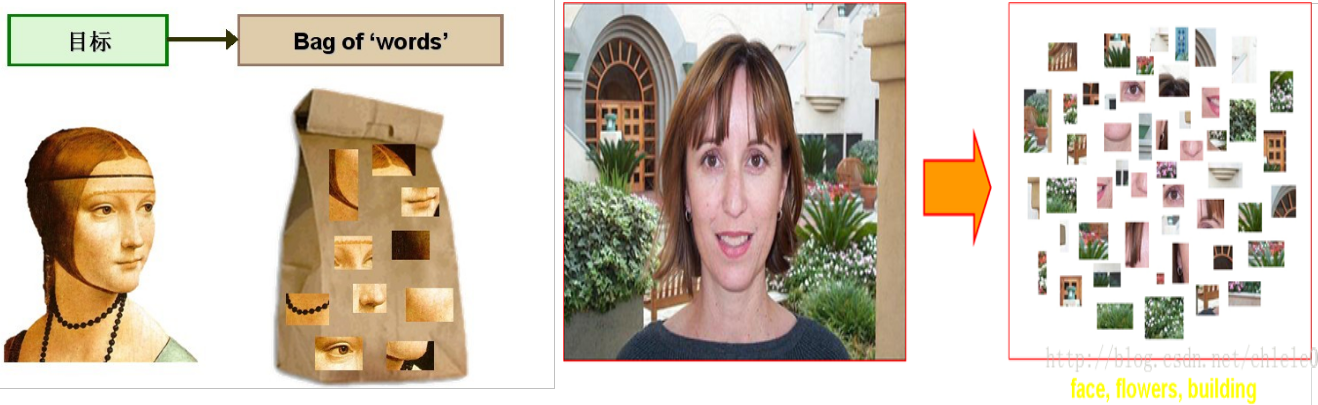- 浅析 Bag of Feature

YOLACT 中的 Prototypes 与 BOFs 类图像特征提取方法的区别是：

 - BOFs 是特征提取方法，其采用 prototypes 来表示特征；而 YOLACT 将 prototypes 用于生成实例分割的 masks.

 - YOLACT 是针对每张图片学习 prototypes，而 BOFs 是对整个数据集所学习的全局共享的 prototypes.

## 2. YOLACT 方法

 - 并行化分支一：采用 FCN 生成图像大小(image-sized)的 prototype masks 集合，其不依赖于任何一个实例.

YOLACT 结构如图：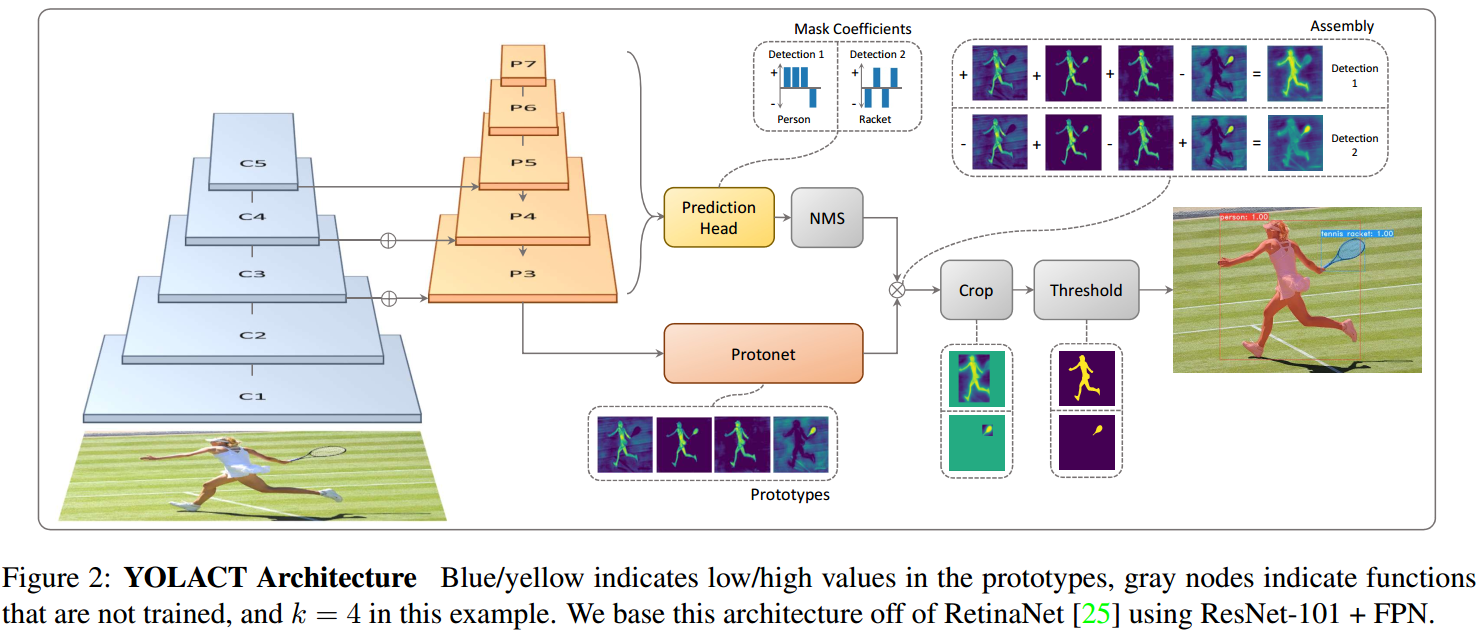### 2.2. Prototype 生成

YOLACT 的 prototype 生成分支(protonet) 会输出整张图片的 k 个 prototype masks 集合.

Protonet 是 FCN 网络，其输出层包含 k 个通道(channels)，每个通道分别表示一个 prototype. 如图Fig3. Protonet 类似于语义分割任务，但其不同之处在于，其没有具体的关于 prototypes 的 loss；所有的监督都来自于线性组合后最终的 mask loss.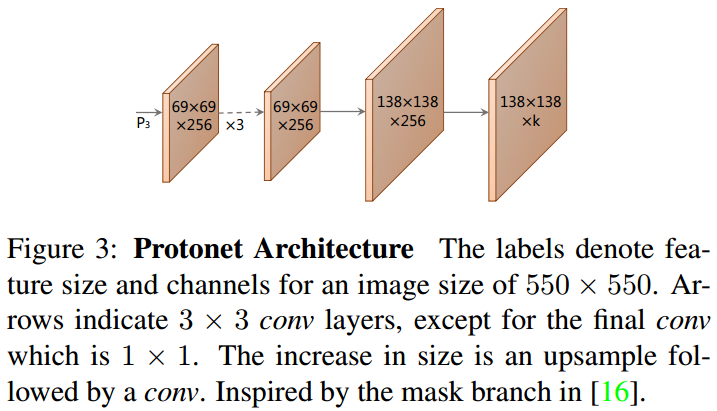- 采用 deeper backbone 特征的 Protonet 能够生成更鲁棒的 masks；

 - 更高分辨率的 prototypes 能够得到更高质量的 masks 以及对于小目标物体具有更好的性能.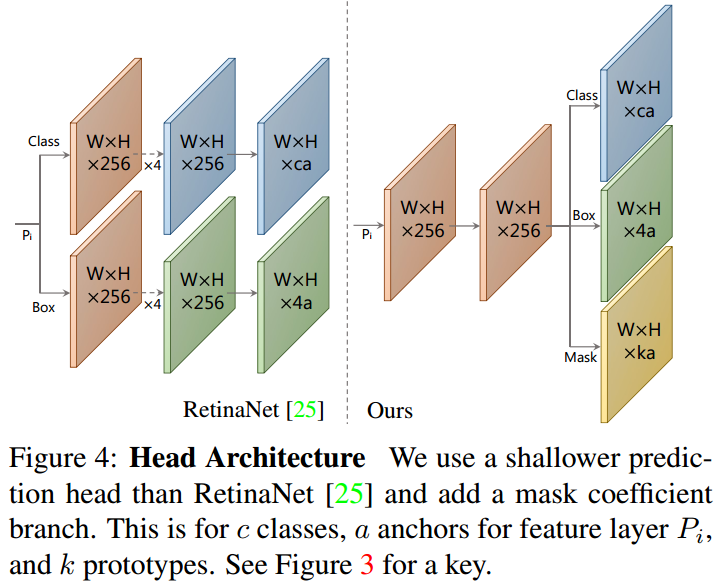$$M = \sigma(PC^T)$$

$C$ 为 $n \times k$ 的 mask coefficients 矩阵；$n$ 为 NMS 和阈值处理后得到的 $n$ 个实例数.

### 2.5. Losses

YOLACT 模型训练时，采用了三个 losses 函数：

 - 分类loss $L_{cls}$

 - 边界框回归 loss $L_{box}$

 - mask loss $L_{mask}$，计算的是组合的 masks $M$ 与 GT masks $M_{gt}$ 之间的逐像素的二值交叉熵：$L_{mask} = BCE(M, M_{gt})$.

### 2.7. Emergent Behavior

YOLACT 在处理实例分割时，出现平移变化(translation variance) 之处仅有采用预测的边界框对最终的 mask 进行裁剪的操作. 然而，实际上，对于中型及大型目标物体，不需要裁剪操作，YOLACT 也是有效的. YOLACT 通过对其 prototypes 的不同激活值，能够在网络自身学习到如何定位实例的能力. 对此，这里如图 Fig 5 例示.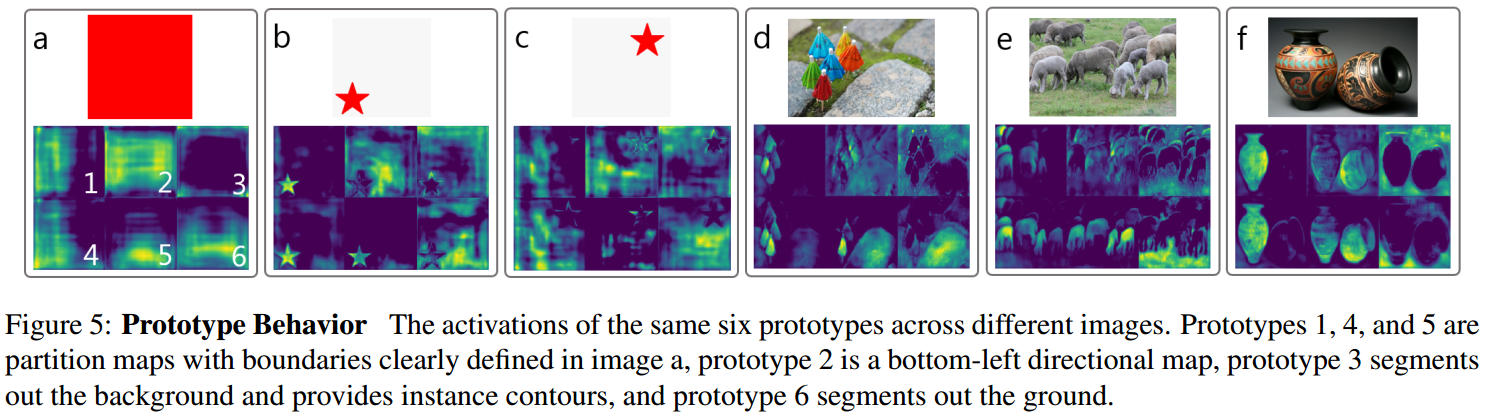Fig 5. 不同图片的相同的六种 prototypes 的激活情况例示. Prototype 1,4和5 是在图片 a 中具有清晰便捷定义的分割图；prototype 2 是 bottom-left 方向图；prototype 3 分割出背景，并给出了实例的轮廓；prototype 6 分割出了地面 (ground).

## 3. Backbone 检测器

### 3.1. YOLACT 检测器

YOLACT 采用 smooth-L1 loss 来训练 box 回归器，并采用与 SSD 相同的方式编码 box 回归坐标值.

YOLACT 采用 softmax 交叉熵 loss 来对 c 个类别标签(positive labels)和 1 个背景类别(background label)进行处理. 采用 OHEM 选择训练样本，$neg:pos=3:1$.

## 4. Fast NMS

YOLACT 中 Fast NMS 可以并行地对每个实例进行判读是保留还是丢弃，其实现如下：

 - 首先根据数据集中 $c$ 个类别中每一类的置信度排名，计算 top n 个检测结果成对间的 IoU，得到 $c \times n \times n$ 的矩阵$X$(对角矩阵).

 - 然后，通过判断是否有置信度较高(higher-scoring)的检测结果，其对应的矩阵 $X$ 中的 IoU 元素值大于设定阈值 $t$，以得到待删除的检测结果. 具体地，

• 首先设置对角矩阵 $X$ 的下三角和对角元素的值为0：

$$X_{kij} = 0, \forall k, j, i \geq j$$

• 然后，逐列求 max，以计算得到由每个检测结果的最大 IoU 值组成的矩阵 $K$：

$$K_{kj} = max _{i} (X_{kij}), \forall k, j$$

• 最后，对矩阵 $K$ 求阈值 $t$，$K < t$，即可得到对于每个类别所保留的检测结果.

## 5. Results

### 5.1. 实例分割